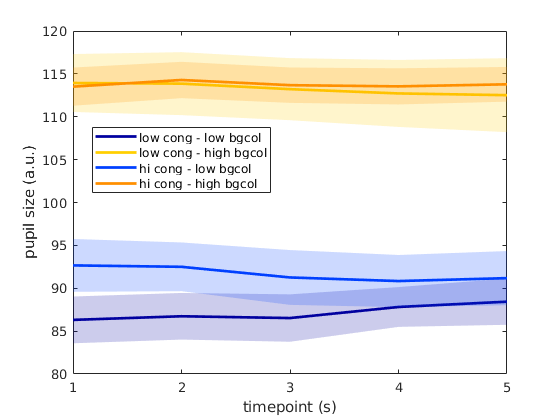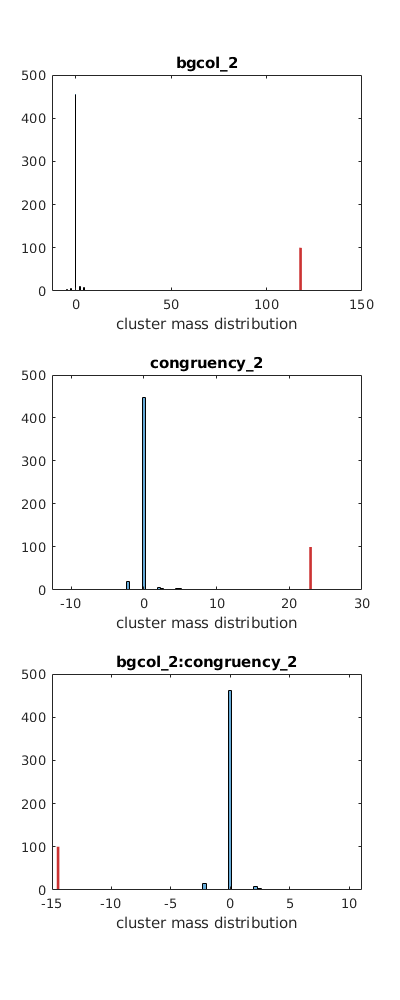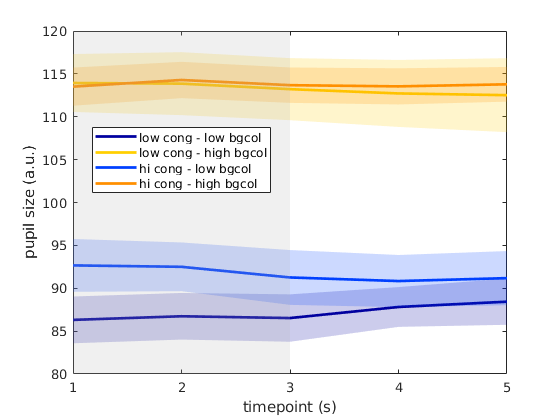## How to use linear mixed effect models (LMMs) with cluster-based permutation test?

(for comments, either resepond to the mailing list or open an issue here: https://github.com/ozika/perm_test_lme)

### Scenario 1:

(I just made these examples up, i tried to keep the dimensions unique in size for identifiability)
Dependent variable (DV)
• pupil size (measured every second after onset, for 5 seconds)
Fixed effects
• background color (within subject, 2 levels)
• congruency (within subject, 2 levels)
Random effects
• participant (10 participants/levels)
Each participant completes 40 trials, 10 one of each kind in the 2x2 design.
The overall DV dataset is therefore 400x5 matrix.
---
Analysis (code below to be specific):
1) Run LMM for each data point using: pupil ~ bgcolor + congruency + bgcolor*congruency + (1|sub)
2) Collect t-values
3) Identify clusters
4) Generate null distribution by shuffling labels of bgcolor and congruency within each subject. Include permutations where no cluster was above threshold and assign them 0 cluster mass.
5) Calcualte desired threshold based on the null distribution
6) Evaluate actual clusters for each effect.

### Scenario 2:

Dependent variable (DV)
• pupil size (measured every second after onset, for 5 seconds)
Fixed effects
• background color (within subject, 2 levels)
• drug/placebo (between subject, 2 levels)
Random effects
• participant (10 participants/levels)
This is the same scenario as above but one factor is now a between subject variable. Would the right randomization strategy be to shuffle bgcolor within subject and then shuffle labels for entire subjects? (e.g. if each sub has 100 sampels altogether, shuffle the entire blocks of 100)
Is there a disadvantage in shuffling ALL variables across all samples/subjects?

## Back to Scenario 1

[GS, Gkey, S, Skey, mle] = get_stats(iv, dv, {'bgcol', 'congruency'});
map = colormap('jet');
col4 = [map(2,:) ; map(12,:); map(43,:); map(47,:)];

## visualize data

f=figure;
for cn = 1:2
for bg = 1:2
idx = (bg-1)*2+cn;
gme = GS(Gkey.bgcol==num2str(bg) & Gkey.congruency == num2str(cn),:,1);
gse = GS(Gkey.bgcol==num2str(bg) & Gkey.congruency == num2str(cn),:,2);
plot(1:numel(gme), gme, 'LineWidth', 2, 'Color', col4(idx,:));
hold on
end
end
t=gca; t.XTick = 1:5;
legend({'low cong - low bgcol','low cong - high bgcol', 'hi cong - low bgcol','hi cong - high bgcol'}, 'location', 'best');
ylabel('pupil size (a.u.)')
xlabel('timepoint (s)');## run LMM for each data point

eq = 'dv ~ bgcol*congruency + (1|id)';
L =[]; labels ={};
for i = 1:size(dv,2)
e = [array2table(dv(:,i), 'VariableNames', {'dv'}) iv];
lme = fitlme(e, eq);
for bb = 2:length(lme.Coefficients.Estimate)
labels{bb-1} = lme.Coefficients.Name{bb};
L.E(bb-1, i) = lme.Coefficients.Estimate(bb);
L.SE(bb-1, i) = lme.Coefficients.SE(bb);
L.T(bb-1, i) = lme.Coefficients.tStat(bb);
L.P(bb-1,i) = lme.Coefficients.pValue(bb);
end
end

## find clusters

tcrit = 2;
for eff = 1:size(L.E,1)
sigT_true = repmat(0, 1, length(L.T(eff,:)));
sigT_true(abs(L.T(eff,:))>tcrit) = 1;
T_true = L.T(eff,:);
disp(labels{eff});
[C_sub{eff}] = clusterize(sigT_true, T_true);
disp(array2table(C_sub{eff}, 'VariableNames', {'cluster_size', 'cluster_mass', 'na', 'cl_start', 'cl_end'}));
end
bgcol_2
cluster_size cluster_mass na cl_start cl_end ____________ ____________ __ ________ ______ 4 117.83 0 1 5
congruency_2
cluster_size cluster_mass na cl_start cl_end ____________ ____________ __ ________ ______ 3 22.926 0 1 4
bgcol_2:congruency_2
cluster_size cluster_mass na cl_start cl_end ____________ ____________ __ ________ ______ 2 -14.493 0 1 3
Ok, so we find one cluster for each effect. As expected there is a significant main effect of background color across all five time points, but there also seems to be a main effect of congruency (time points 1 - 4) and an interaction at time points 1 - 3.

## permutation test

This is the part that I am not sure about. Intuitively, since both fixed effects are within subject I'd permute the labels within each participant. (The alternative is across all participants).
noperms = 500;
fxeff = {'bgcol', 'congruency'};
rndeff = {'id'};
ids = unique(iv.id);
run = 0;
if run
C_perm = cell(1,size(L.E,1));
for perms = 1:noperms
% shuffle labels of all independent variables
tiv = table; %permutation-specific independent vars
vars = [fxeff];
for sub = 1:numel(ids) %loop over participants
chunk = iv(ismember(iv.id, ids(sub)),:);
for eff = 1:size(vars,2) % loop over vars
chunk.(vars{eff}) = chunk.(vars{eff})(randperm(length(chunk.(vars{eff}))));
end
tiv = [tiv;chunk];
end
TT=[]; H=[];
for i = 1:size(dv,2)
e = [array2table(dv(:,i), 'VariableNames', {'dv'}) tiv];
lme = fitlme(e, eq); %TT=[];
for bb = 2:length(lme.Coefficients.Estimate)
TT(bb-1, i) = lme.Coefficients.tStat(bb);
if abs(TT(bb-1, i)) > tcrit
H(bb-1,i) =1;
else
H(bb-1,i) =0;
end
end
end
for var = 1:size(TT,1) % loop over regressors
%take cluster with highest cluster mass
cltemp = []; cltemp = clusterize(H(var,:), TT(var,:));
maxclust =[];
if isempty(cltemp)
maxclust = repmat(0,1,5); %if no significnat cluster, record cluster with cluster mass 0
else
%otherwise take biggest cluster
maxclust = cltemp(find(abs(cltemp(:,2))==max(abs(cltemp(:,2)))),:);
end
C_perm{var} = [C_perm{var}; maxclust];
end
end
save('../data/dataset1_perm_data.mat');
else
end

## show null distributions and actual cluster mass (red)

%% Get distribution for each variable
distr =[];
P ={};
alpha =0.05;
f=figure;
for eff = 1:size(L.E,1)
% permutation distribution T-based cluster mass
C=[];
C = C_perm{eff};
C_true = C_sub{eff};
distr = sort(C(:,2));
n = length(distr);
low_perc = (alpha/2)*n;
hi_perc = (1-(alpha/2))*n;
low_val = distr(round(low_perc));
hi_val = distr(round(hi_perc));
%%% back to real data: assess each cluster
for c = 1:size(C_true)
if C_true(c,2) > hi_val || C_true(c,2) < low_val
C_true(c,3) = 1;
else
C_true(c,3) = 0;
end
end
P{eff}.name = lme.Coefficients.Name{eff+1};
P{eff}.clusters = C_true;
P{eff}.perm_distribution = distr;
subplot(size(L.E,1),1, eff);
histogram(P{eff}.perm_distribution, 50); hold on;
xlabel('cluster mass distribution');
plot(repmat(P{eff}.clusters(1,2), 2, 1), [0 100], 'Color', [0.8 0.2 0.2], 'LineWidth', 2);
title(P{eff}.name);
end
f.Position(3) = 400;
f.Position(4) = 1000;This doesn't seem right to me, but it could be only becuse the data are artificially generated.

## plot again with significant interaction cluster(s)

f=figure;
for cn = 1:2
for bg = 1:2
idx = (bg-1)*2+cn;
gme = GS(Gkey.bgcol==num2str(bg) & Gkey.congruency == num2str(cn),:,1);
gse = GS(Gkey.bgcol==num2str(bg) & Gkey.congruency == num2str(cn),:,2);
plot(1:numel(gme), gme, 'LineWidth', 2, 'Color', col4(idx,:));
hold on